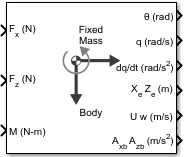# 3DOF (Body Axes)

Implement three-degrees-of-freedom equations of motion with respect to body axes

## Library

Equations of Motion/3DOF

•## Description

The 3DOF (Body Axes) block considers the rotation in the vertical plane of a body-fixed coordinate frame about a flat Earth reference frame.The equations of motion are

`$\begin{array}{l}{A}_{xb}=\stackrel{˙}{u}=\frac{{F}_{x}}{m}-qw-g\mathrm{sin}\theta ,{A}_{xe}=\frac{{F}_{x}}{m}-\epsilon \mathrm{sin}\theta \\ {A}_{zb}=\stackrel{˙}{w}=\frac{{F}_{z}}{m}+qu+g\mathrm{cos}\theta ,{A}_{ze}=\frac{{F}_{z}}{m}+\epsilon \mathrm{cos}\theta \\ \stackrel{˙}{q}=\frac{M}{{I}_{yy}}\\ \stackrel{˙}{\theta }=q\end{array}$`

where the applied forces are assumed to act at the center of gravity of the body.

## Parameters

### Main

Units

Specifies the input and output units:

Units

Forces

Moment

Acceleration

Velocity

Position

Mass

Inertia

`Metric (MKS)`

Newton

Newton-
meter

Meters per second squared

Meters per second

Meters

Kilogram

Kilogram meter squared

```English (Velocity in ft/s)```

Pound

Foot- pound

Feet per second squared

Feet per second

Feet

Slug

Slug foot squared

```English (Velocity in kts)```

Pound

Foot- pound

Feet per second squared

Knots

Feet

Slug

Slug foot squared

Mass Type

Select the type of mass to use:

 `Fixed` Mass is constant throughout the simulation. `Simple Variable` Mass and inertia vary linearly as a function of mass rate. `Custom Variable` Mass and inertia variations are customizable.

The `Fixed` selection conforms to the previously described equations of motion.

Initial velocity

A scalar value for the initial velocity of the body, (V0).

Initial body attitude

A scalar value for the initial pitch attitude of the body, (θ0).

Initial incidence

A scalar value for the initial angle between the velocity vector and the body, (α0).

Initial body rotation rate

A scalar value for the initial body rotation rate, (q0).

Initial position (x,z)

A two-element vector containing the initial location of the body in the flat Earth reference frame.

Initial Mass

A scalar value for the mass of the body.

Inertia

A scalar value for the inertia of the body.

Gravity Source

Specify source of gravity:

 `External` Variable gravity input to block `Internal` Constant gravity specified in mask
Acceleration due to gravity

A scalar value for the acceleration due to gravity used if internal gravity source is selected. If gravity is to be neglected in the simulation, this value can be set to 0.

Include inertial acceleration

Select this check box to enable an additional output port for the accelerations in body-fixed axes with respect to the inertial frame. You typically connect this signal to the accelerometer.

### State Attributes

Assign unique name to each state. You can use state names instead of block paths during linearization.

• To assign a name to a single state, enter a unique name between quotes, for example, `'velocity'`.

• To assign names to multiple states, enter a comma-delimited list surrounded by braces, for example, `{'a', 'b', 'c'}`. Each name must be unique.

• If a parameter is empty (`' '`), no name assignment occurs.

• The state names apply only to the selected block with the name parameter.

• The number of states must divide evenly among the number of state names.

• You can specify fewer names than states, but you cannot specify more names than states.

For example, you can specify two names in a system with four states. The first name applies to the first two states and the second name to the last two states.

• To assign state names with a variable in the MATLAB® workspace, enter the variable without quotes. A variable can be a character vector, cell array, or structure.

Velocity: e.g., {'u', 'w'}

Specify velocity state names.

Default value is `''`.

Pitch attitude: e.g., 'theta'

Specify pitch attitude state name.

Default value is `''`.

Position: e.g., {'Xe', 'Ze'}

Specify position state names.

Default value is `''`.

Pitch angular rate: e.g., 'q'

Specify pitch angular rate state name.

Default value is `''`.

## Inputs and Outputs

InputDimension TypeDescription

First

Contains the force acting along the body x-axis, (Fx).

Second

Contains the force acting along the body z-axis, (Fz).

Third

Contains the applied pitch moment, (M).

Fourth (Optional)

Contains the block is gravity in the selected units.
OutputDimension TypeDescription

First

Contains the pitch attitude, within ±pi, in radians (θ).

Second

Contains the pitch angular rate, in radians per second (q).

Third

Contains the pitch angular acceleration, in radians per second squared $\left(\stackrel{˙}{q}\right)$.

Fourth

Two-element vectorContains the location of the body, in the flat Earth reference frame, (Xe, Ze).

Fifth

Two-element vectorContains the velocity of the body resolved into the body-fixed coordinate frame, (u, w).

Sixth

Two-element vectorContains the acceleration of the body resolved into the body-fixed coordinate frame, (Ax, Az).

Seventh

Two-element vectorContains the accelerations in body-fixed axes with respect to inertial frame (flat Earth). You typically connect this signal to the accelerometer.

## Examples

See `aero_guidance` for an example of this block.

## Extended Capabilities

### C/C++ Code GenerationGenerate C and C++ code using Simulink® Coder™.

#### Introduced in R2006a

##### Support